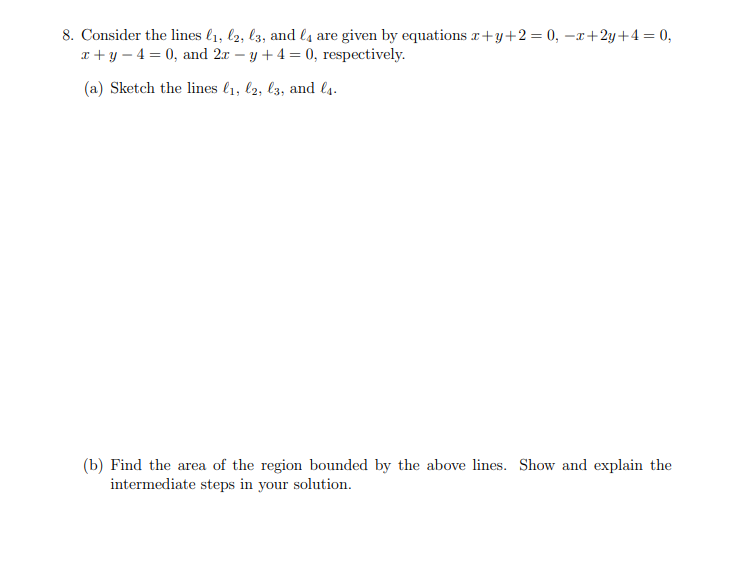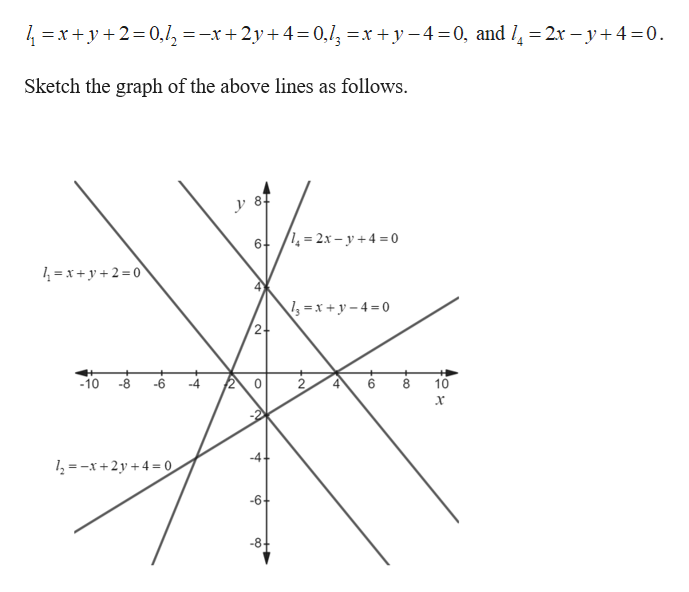# 8. Consider the lines l1, l2, l3, and ls are given by equations r+y2 = 0, -x+2y+4 = 0,y40, and 2r -y4 0, respectively(a) Sketch the lines , l2, l3, and l4(b) Find the area of the region bounded by the above lines. Show and explain theintermediate steps in your solution

Question
116 views

Practice Question 8.3help_outlineImage Transcriptionclose8. Consider the lines l1, l2, l3, and ls are given by equations r+y2 = 0, -x+2y+4 = 0, y40, and 2r -y4 0, respectively (a) Sketch the lines , l2, l3, and l4 (b) Find the area of the region bounded by the above lines. Show and explain the intermediate steps in your solution fullscreen
check_circle

Step 1

(a) The given li...help_outlineImage Transcriptionclose01 x +y-40, and / 2r - y4=0 4 x+y2 0,=-x+2y4 Sketch the graph of the above lines as follows. y 8 42x-y4 6 x+y2 0 8 10 0 -8 -6 2 10 -4 2=x+2y +4= 0 -6- -8 fullscreen

### Want to see the full answer?

See Solution

#### Want to see this answer and more?

Solutions are written by subject experts who are available 24/7. Questions are typically answered within 1 hour.*

See Solution
*Response times may vary by subject and question.
Tagged in
MathAlgebra

### Other